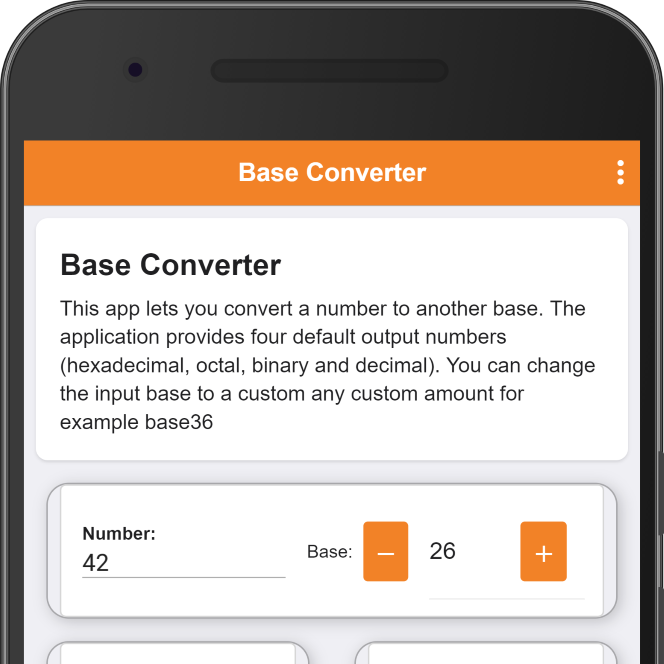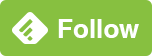## Base Converter

This simple simple conversion tool converts the same number between different number systems. You can convert from any base, for example, hexadecimal, decimal, binary, octal, up to base 36, which is the largest base you can represent using letters, and numbers (0-9 + A-Z).# Base Converter

An easy to use application which converts to different numerical systems.

You can also find it in the play store.

For example: If we convert the decimal number “123456789” to base 16 (hexadecimal) we get: “75bcd15”, octal is “726746425” and with binary we get “111010110111100110100010101”. You can use the tool to automate or simplify your work.

Also, checkout simple function plotter which allows you to plot, visualize and save algebraic formula’s.